# Fill in the blanks 1 has only two

• Slides: 11Fill in the blanks: (1) _____ has only two possible values 0 and 1. (2) There are _____bits in a byte. (3) 1 kilobyte of memory space can store _____ bites. (4) 1 gigabyte = ______ megabytes. (5) Using only 7 bits initially, the number of printing and nonprinting characters that ASCII code could represent was _____. (6) The decimal value of the space character in the ASCII code is _____. (7) In the ASCII code, the decimal values of the capital letters of the English alphabet range from ______ to ______.Fill in the blanks: (1) Binary number system has only two possible values 0 and 1. (2) There are 8 bits in a byte. (3) 1 kilobyte of memory space can store 8192 bites. (4) 1 gigabyte = 1024 megabytes. (5) Using only 7 bits initially, the number of printing and nonprinting characters that ASCII code could represent was 128. (6) The decimal value of the space character in the ASCII code is 32. (7) In the ASCII code, the decimal values of the capital letters of the English alphabet range from 65 to 90.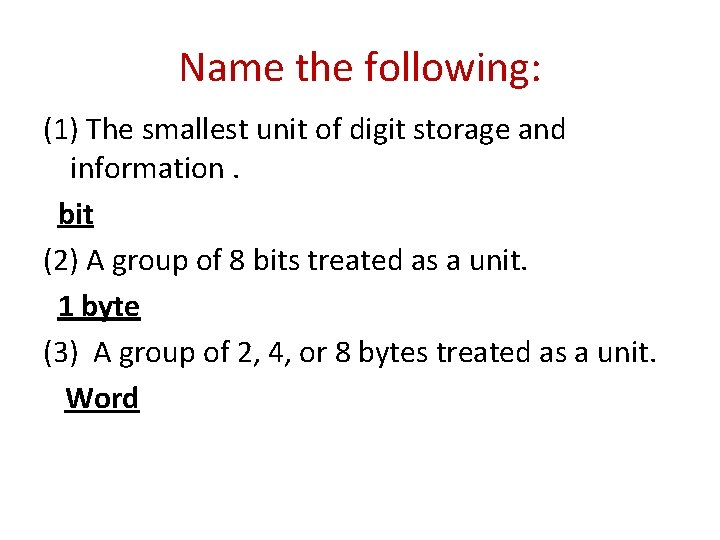Name the following: (1) The smallest unit of digit storage and information. bit (2) A group of 8 bits treated as a unit. 1 byte (3) A group of 2, 4, or 8 bytes treated as a unit. Word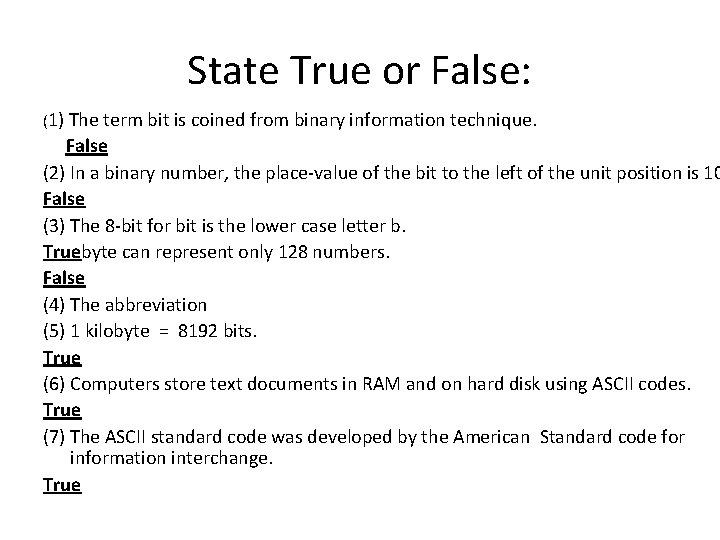State True or False: (1) The term bit is coined from binary information technique. False (2) In a binary number, the place-value of the bit to the left of the unit position is 10 False (3) The 8 -bit for bit is the lower case letter b. Truebyte can represent only 128 numbers. False (4) The abbreviation (5) 1 kilobyte = 8192 bits. True (6) Computers store text documents in RAM and on hard disk using ASCII codes. True (7) The ASCII standard code was developed by the American Standard code for information interchange. TrueWhat is the decimal system? Ans: The number system with base 10 is called decimal system.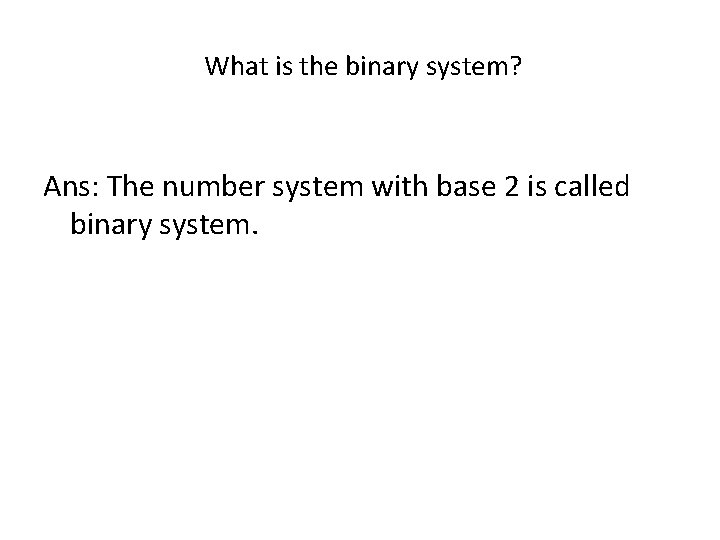What is the binary system? Ans: The number system with base 2 is called binary system.Write any two points of difference between the decimal and binary number systems. Decimal number system 1) The number system with base 10 is called decimal system. Binary number system 1)The number system with base 2 is called binary system. 2) In decimal number system each 2) In binary number system each of 10 digits are 0, 1, 2, 3, 4, 5, 6, 7, 8, 9. of 2 digits are 0, 1.What is the place-value of each bit in the binary notation? Ans: In the binary notation, the place-value of each successive bit to the left of the unit position indicates a multiple of successive power of 2.What is the byte? Ans: A group of 8 bit treated as a unit by computer hardware is called by a byte.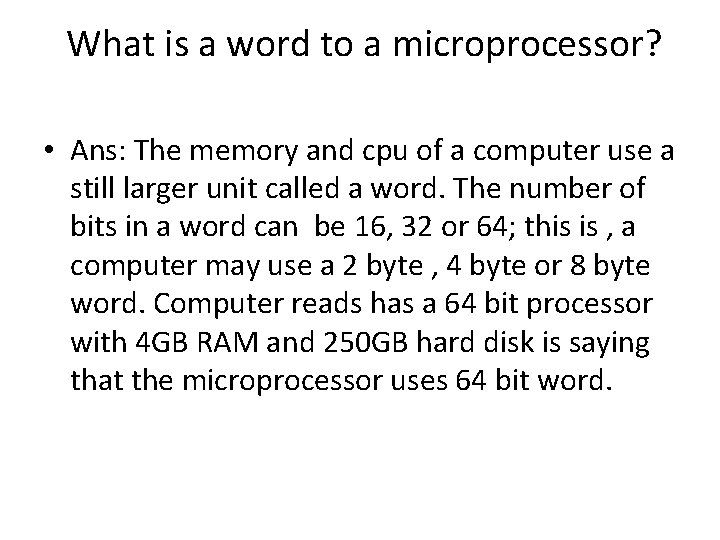What is a word to a microprocessor? • Ans: The memory and cpu of a computer use a still larger unit called a word. The number of bits in a word can be 16, 32 or 64; this is , a computer may use a 2 byte , 4 byte or 8 byte word. Computer reads has a 64 bit processor with 4 GB RAM and 250 GB hard disk is saying that the microprocessor uses 64 bit word.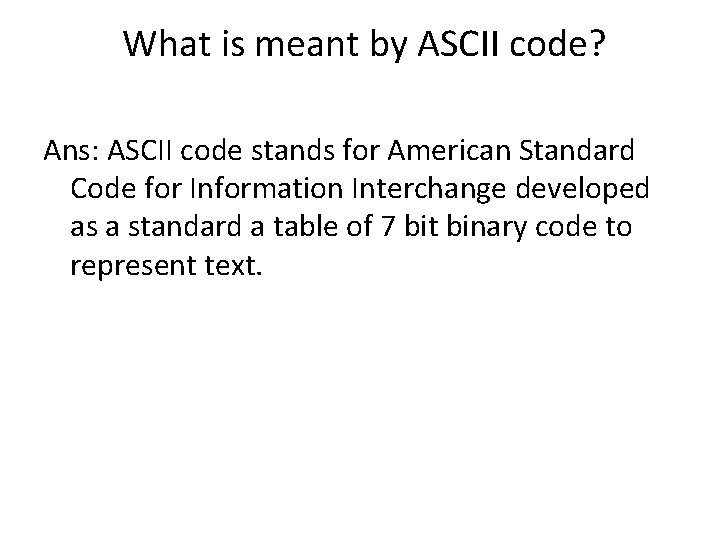What is meant by ASCII code? Ans: ASCII code stands for American Standard Code for Information Interchange developed as a standard a table of 7 bit binary code to represent text.